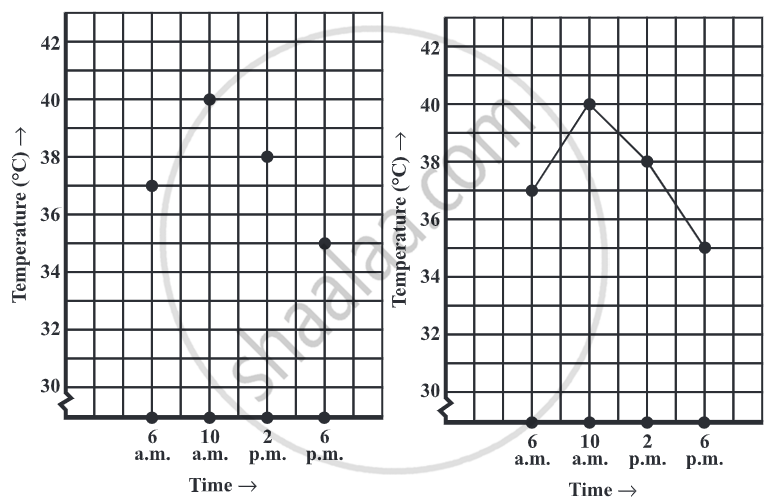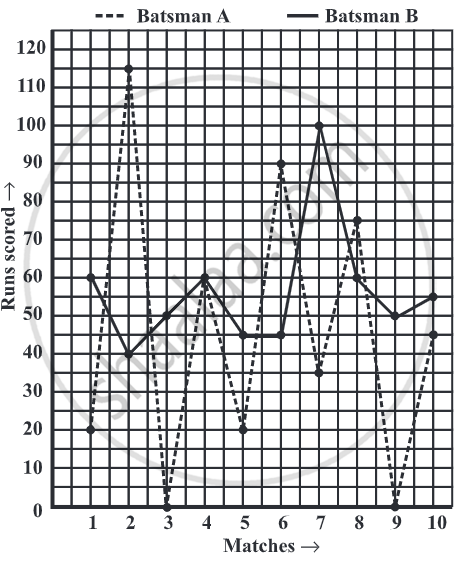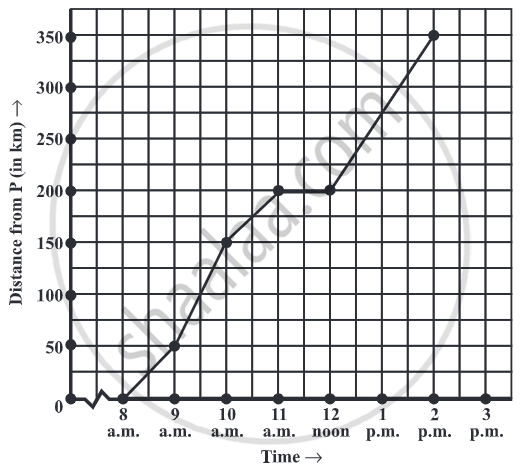# Concept of a Line Graph

## Definition

Line graph: A line graph displays data that changes continuously over periods of time.

## Notes

### Concept of a Line Graph:

A line graph displays data that changes continuously over periods of time.

1. Consider the following data, given in tabular form.

 Time 6 a.m. 10 a.m. 2 p.m. 6 p.m. Temperature(°C) 37 40 38 35• The horizontal line (usually called the x-axis) shows the timings at which the temperatures were recorded.

• Temperature(°C) is labelled on the vertical line (usually called the y-axis).

• By this line graph, we can easily understand the increase and decrease of temperature over the course of 12 hours from 6 AM to 6 PM.

#### Interpretation of line graph:

1) The given graph represents the total runs scored by two batsmen A and B, during each of the ten different matches in the year 2007. Study the graph and answer the following questions.(i) What information is given on the two axes?
(ii) Which line shows the runs scored by batsman A?
(iii) Were the run scored by them the same in any match in 2007? If so, in which match?
(iv) Among the two batsmen, who is steadier? How do you judge it?

### Solution:

(i) The horizontal axis (or the x-axis) indicates the matches played during the year 2007. The vertical axis (or the y-axis) shows the total runs scored in each match.

(ii) The dotted line shows the runs scored by Batsman A. (This is already indicated at the top of the graph).

(iii) During the 4th match, both have scored the same number of 60 runs. (This is indicated by the point at which both graphs meet).

(iv) Batsman A has one great “peak” but many deep “valleys”. He does not appear to be consistent. B, on the other hand, has never scored below a total of 40 runs, even though his highest score is only 100 in comparison to 1 15 of A. Also, A has scored a zero in two matches, and in a total of 5 matches, he has scored less than 40 runs. Since A has a lot of ups and downs, B is a more consistent and reliable batsman.

## Example

The given graph describes the distances of a car from a city P at different times when it is travelling from City P to City Q, which are 350 km apart. Study the graph and answer the following:(i) What information is given on the two axes?
(ii) From where and when did the car begin its journey?
(iii) How far did the car go in the first hour?
(iv) How far did the car go during (i) the 2nd hour? (ii) the 3rd hour?
(v) Was the speed same during the first three hours? How do you know it?
(vi) Did the car stop for some duration at any place? Justify your answer.
(vii) When did the car reach City Q?
(i) The horizontal (x) axis shows the time. The vertical (y) axis shows the distance of the car from City P.
(ii) The car started from City P at 8 a.m.
(iii) The car travelled 50 km during the first hour.
(iv) The distance covered by the car during
(a) the 2nd hour (i.e., from 9 am to 10 am) is 100 km, (150 – 50).
(b) the 3rd hour (i.e., from 10 am to 11 am) is 50 km (200 – 150).
(v) From the answers to questions (iii) and (iv), we find that the speed of the car was not the same all the time. (In fact, the graph illustrates how the speed varied).
(vi) We find that the car was 200 km away from city P when the time was 11 a.m. and
also at 12 noon. This shows that the car did not travel during the interval of 11 a.m. to 12 noon. The horizontal line segment representing “travel” during this period is illustrative of this fact.
(vii) The car reached City Q at 2 p.m.
If you would like to contribute notes or other learning material, please submit them using the button below.